Open In App
Related Articles
• CBSE Class 10 Maths Notes
• CBSE Class 10 Maths Formulas
• NCERT Solutions for Class 10 Maths
• RD Sharma Class 10 Solutions

# Fundamental Theorem of Arithmetic

Arithmetic is the game of numbers and each and every number is divided into some or other group, For instance: there exists composite numbers, even numbers, odd numbers, and prime numbers. Prime numbers are one of those that can be a part of each and every number, If a number is broken down into smaller numbers, the smallest numbers to exist being a part of that number are nothing but prime numbers.

### Prime Numbers

Prime numbers are the numbers that have only and only 2 factors. They are 1 and the number itself. For example: 2,3,5,7,11,13 and so on.

All natural numbers can be written as a product of their prime factors. For example: 24 = 2 × 3 × 2 × 2 or 13 = 13 × 1 and so on. Can we say that the vice-versa is also true? Can any natural number be obtained by multiplying prime numbers? This question is answered by The Fundamental Theorem of Arithmetic, also known as the Unique prime factorization theorem. The major significance of the Fundamental theorem of arithmetic is that it tells about the uniqueness of prime factorization.

## Fundamental Theorem of Arithmetic

Let’s take some set of prime numbers, for example — {3, 2, 7}. How many numbers do you think we can make from their multiplication? 3 × 2 = 6, 3 × 3 × 2 = 18, 7 × 2 = 14 and so on. So, we can say, infinitely many numbers can be made from these prime numbers. But does that prove that we can generate every possible number?

Yes, there are infinitely many possible prime numbers and from their multiplication, we can generate infinite numbers and that is the crux of the Fundamental Theorem of Arithmetic. To further develop this concept let’s look at the factorization of a number. Suppose we are given a number x = 36.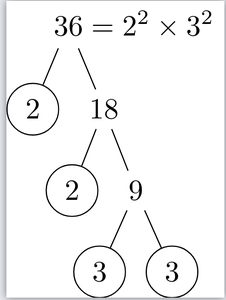The figure above represents the factorization tree of the number. 36 = 2 × 2 × 3 × 3. It is a product of prime numbers. If we keep on trying different numbers, we see that all the numbers can be represented as product primes. In a more formal way,

Theorem:

Every composite number can be expressed (factorized) as a product of primes, and this factorization is unique, apart from the order in which the prime factors occur.

This is called Fundamental Theorem of Arithmetic.

This theorem says that every composite number can be rewritten as the product of prime numbers in a “unique” way, except for the order in which primes occur.

### Proof of Fundamental Theorem of Arithmetic

Step 1: The existence of prime factors, we will prove it b y induction

Firstly, consider n>1

Therefore Initially, n=2. Since n=2 and 2 is a prime number, the result is true.

Consider n>2  (Induction hypothesis: Let result be true for all positive numbers less than n )

No, we will prove that the result is also true for n.

• If n is prime, then n is a product of primes is trivially true.
• If n is not prime i.e n is a composite number, then

n = ab, a, b < n

By induction method, the result is true for a and b (because a<n and b<n). Therefore, by the induction hypothesis, a must be the product of prime numbers and b is a product of prime numbers. Therefore, n = ab is a product of prime numbers. Thus, it is proved by induction.

Step 2: Uniqueness (of factors up to order)

Let n = p1 p2 p3….pk (where p1 p2 p3 …pk are primes)

Let if possible, there be two representations of n as a product of primes

i.e let if possible n=p1 p2 p3….pk = q1 q2 q3 …qr      where pi’s and qj’s are prime numbers

(we will prove that pi’s are the same as qj’s )

Now p1/p1 p2 p3…pk, Therefore, p1/q1 q2 q3…qr (because p1 p2 p3….pk = q1 q2 q3 ….qr)

Therefore, by result p1 must be one of the qj’s.

Let p1 = q1

So we get p1 p2 p3….pk = q1 q2 q3 …qr

= p1 q2 q3…qr

And by cancellation p1 from both sides,

p2 p3 … pk  = q2 q3 …. qr

So by the same argument, we will get p2=q2  and so on.

Thus, n can be expressed as a product of primes uniquely (except for the order)

Hence proved.

Question 1: Factorize the number “4072” and represent it in tree form.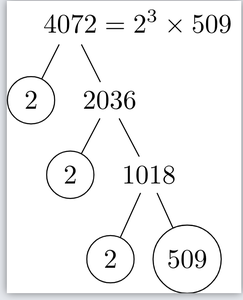Question 2: Factorize the number “324” and represent it in tree form.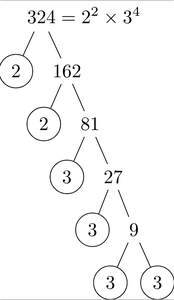Question 3: Factorize the number “16048” and represent it in tree-form.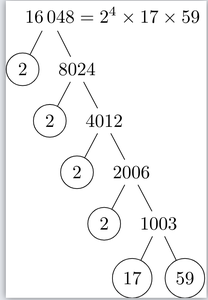### LCM and HCF using Fundamental Theorem of Arithmetic

• HCF known as the highest common factor is the greatest number that divides each of the two numbers given.
• LCM is the lowest common multiple that is the product of all the common prime factors but with their highest degrees/powers.

For example:

Question 1: Find the LCM and HCF of 24 and 36.

Solution:

The Prime factors of 24 = 2× 2×2×3

The prime factors of 36 = 2×2×3×3

HCF = 2×2×2×3, 2×2×3×3 = 2×2×3 = 12

LCM =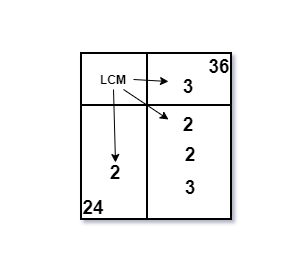2×2×2×3×3 = 72

LCM and HCF can be found with the help of prime factorization too, lets’s look at some examples.

Question 2: Find the LCM and HCF of numbers 6 and 20.

Prime Factorization of 6 can be represented in the following way,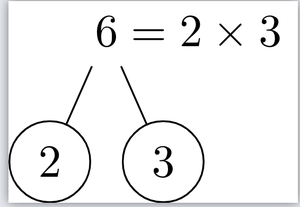Prime Factorization of 20 can be represented in the following way,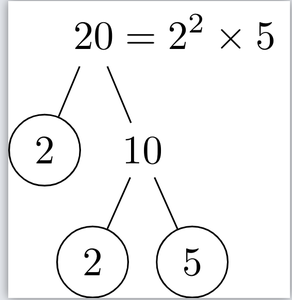So, now we have prime factorization of both the numbers,

6 = 2 × 3

20 = 2 × 2 × 5

We know that

HCF = Product of the smallest power of each common prime factor in the numbers.

LCM = Product of the greatest power of each prime factor, involved in the numbers.

So, HCF(6,20) = 21

LCM(6,20) = 22 × 31 × 5

Question 3: Find the LCM and HCF of numbers 24 and 36.

Prime Factorization of 24: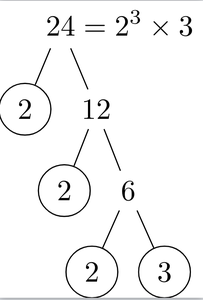Prime Factorization of 36: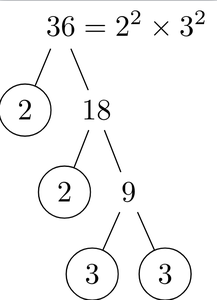24 = 23 × 3 and 36 = 22 × 32

Based on the previous definitions,

HCF(24, 36) = 12

LCM(24, 36) = 72

Fact: In the above examples, notice that for any two numbers “a” and “b”. HCF × LCM = a × b.

Question 4: Suppose that for two numbers “a” and “b”. HCF is given which is 120 and the product of the two numbers is given as 3600. Find the LCM of the two numbers.

Given two numbers “a” and “b”.

LCM(a, b) is unknown while HCF(a, b) = 120 and a × b = 3600.

From the property studied above,

HCF(a, b) × LCM(a, b) = a × b

Plugging in the given values.

120 × LCM(a, b) = 3600

LCM(a, b) = 30